# Computing a smooth circular notch

Last update: 02/22/2018, Amir Hardon, Flutter.

Our objective is to compute a path segment for a notch in a top edge of a container as shown below.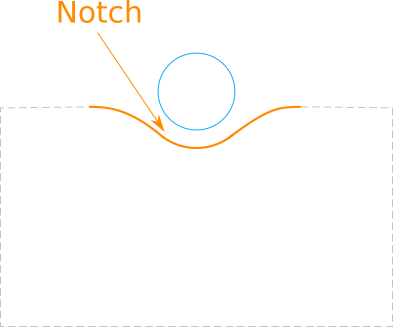The notch path is composed of 3 segments: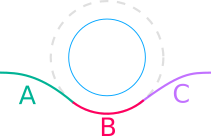• Segment B is an arc on the dashed circle.
• Segments A and C are Bezier curves showing a smooth transition from a straight line (the container’s top edge) to arc B.

The radius for the “guest” circle is denoted GR.

The minimal margin between the “guest” circle and the notch is a parameter notchMargin.

Thus segment B is an arc on a circle with radius r = GR + notchMargin with the same center as the “guest” circle (this is the dashed circle in the diagram above).

For the computations below we set the origin of a cartesian coordinates system at the center of the “guest” circle.

### Finding the control points for segment A’s bezier curve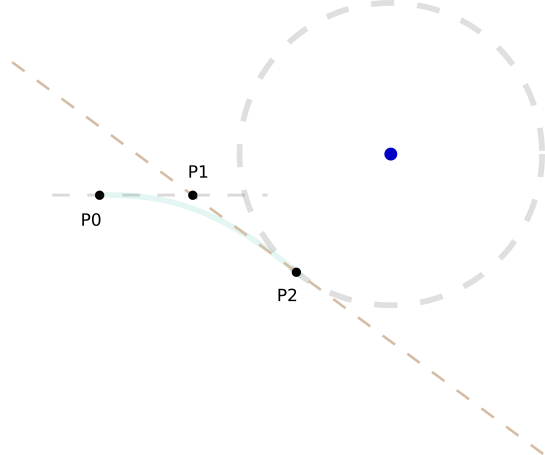(explaining for segment A, segment C is symmetric)

Segment A connects a point on the top edge of the container (P0), with the intersection point of segments A and B (P2).

We want the segment connections at P0 and P2 to be “smooth”.

By “smooth” we mean the tangents at the connection points are the same for the 2 connected segments (or the derivatives to be equal at the connection points).

We use a quadratic bezier curve - Given P0 and P2, we are looking for a curve that has the same derivative as the circle at P2, and has a derivative of 0 at P0.

We will use a quadratic bezier curve as it has just the degrees of freedom that lets us impose these requirements.

Given the container’s top edge and the circle, we find P2.

We set 2 parameters which can be used as knobs to compute the segment’s curvature:

• s1 - is the distance of P0 from the bounding square of the circle.
• s2 - is the distance of P1 from the bounding square of the circle.

With these parameters we know P0 and P2, and we are now looking for P2:

The requirements for P2 are:

• P2 is on the circle’s circumference.
• The line that goes through P1 and P2 is tangent to the circle at P2.

This gives us the following set of equations:

•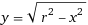(P2 is on the circle’s circumference)
•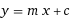(P2 is on a line L)
•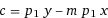(line L passes through p1)
• At x = P2x,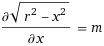<==>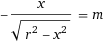(the slope of L equals the slope of the circle at point P2)

Solving for x gives: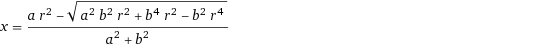,(P1x=a, P1y=b)

There are 2 solutions as there are 2 P2s that satisfy our requirements, one in the top half of the circle and one in the bottom half, we always select the solution that’s in the bottom half.